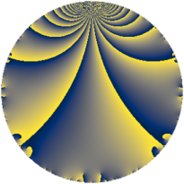# Properties

 Label 8034.2.a.mLevel $8034$ Weight $2$ Character orbit 8034.a Self dual yes Analytic conductor $64.152$ Analytic rank $1$ Dimension $2$ CM no Inner twists $1$

# Related objects

## Newspace parameters

 Level: $$N$$ $$=$$ $$8034 = 2 \cdot 3 \cdot 13 \cdot 103$$ Weight: $$k$$ $$=$$ $$2$$ Character orbit: $$[\chi]$$ $$=$$ 8034.a (trivial)

## Newform invariants

 Self dual: yes Analytic conductor: $$64.1518129839$$ Analytic rank: $$1$$ Dimension: $$2$$ Coefficient field: $$\Q(\sqrt{65})$$ Defining polynomial: $$x^{2} - x - 16$$ Coefficient ring: $$\Z[a_1, \ldots, a_{17}]$$ Coefficient ring index: $$1$$ Twist minimal: yes Fricke sign: $$1$$ Sato-Tate group: $\mathrm{SU}(2)$

## $q$-expansion

Coefficients of the $$q$$-expansion are expressed in terms of $$\beta = \frac{1}{2}(1 + \sqrt{65})$$. We also show the integral $$q$$-expansion of the trace form.

 $$f(q)$$ $$=$$ $$q - q^{2} - q^{3} + q^{4} + q^{6} + q^{7} - q^{8} + q^{9} +O(q^{10})$$ $$q - q^{2} - q^{3} + q^{4} + q^{6} + q^{7} - q^{8} + q^{9} + 3 q^{11} - q^{12} + q^{13} - q^{14} + q^{16} + ( 2 - \beta ) q^{17} - q^{18} - q^{21} -3 q^{22} + ( -3 + \beta ) q^{23} + q^{24} -5 q^{25} - q^{26} - q^{27} + q^{28} + 4 q^{31} - q^{32} -3 q^{33} + ( -2 + \beta ) q^{34} + q^{36} + ( -3 - \beta ) q^{37} - q^{39} + ( -1 + \beta ) q^{41} + q^{42} + ( -1 + \beta ) q^{43} + 3 q^{44} + ( 3 - \beta ) q^{46} + ( -5 + \beta ) q^{47} - q^{48} -6 q^{49} + 5 q^{50} + ( -2 + \beta ) q^{51} + q^{52} + ( 2 - \beta ) q^{53} + q^{54} - q^{56} -2 \beta q^{59} + ( -7 + \beta ) q^{61} -4 q^{62} + q^{63} + q^{64} + 3 q^{66} + ( -8 - \beta ) q^{67} + ( 2 - \beta ) q^{68} + ( 3 - \beta ) q^{69} + ( -8 + 2 \beta ) q^{71} - q^{72} + ( -6 + 3 \beta ) q^{73} + ( 3 + \beta ) q^{74} + 5 q^{75} + 3 q^{77} + q^{78} -2 \beta q^{79} + q^{81} + ( 1 - \beta ) q^{82} + 2 \beta q^{83} - q^{84} + ( 1 - \beta ) q^{86} -3 q^{88} + ( -4 - 2 \beta ) q^{89} + q^{91} + ( -3 + \beta ) q^{92} -4 q^{93} + ( 5 - \beta ) q^{94} + q^{96} -4 \beta q^{97} + 6 q^{98} + 3 q^{99} +O(q^{100})$$ $$\operatorname{Tr}(f)(q)$$ $$=$$ $$2q - 2q^{2} - 2q^{3} + 2q^{4} + 2q^{6} + 2q^{7} - 2q^{8} + 2q^{9} + O(q^{10})$$ $$2q - 2q^{2} - 2q^{3} + 2q^{4} + 2q^{6} + 2q^{7} - 2q^{8} + 2q^{9} + 6q^{11} - 2q^{12} + 2q^{13} - 2q^{14} + 2q^{16} + 3q^{17} - 2q^{18} - 2q^{21} - 6q^{22} - 5q^{23} + 2q^{24} - 10q^{25} - 2q^{26} - 2q^{27} + 2q^{28} + 8q^{31} - 2q^{32} - 6q^{33} - 3q^{34} + 2q^{36} - 7q^{37} - 2q^{39} - q^{41} + 2q^{42} - q^{43} + 6q^{44} + 5q^{46} - 9q^{47} - 2q^{48} - 12q^{49} + 10q^{50} - 3q^{51} + 2q^{52} + 3q^{53} + 2q^{54} - 2q^{56} - 2q^{59} - 13q^{61} - 8q^{62} + 2q^{63} + 2q^{64} + 6q^{66} - 17q^{67} + 3q^{68} + 5q^{69} - 14q^{71} - 2q^{72} - 9q^{73} + 7q^{74} + 10q^{75} + 6q^{77} + 2q^{78} - 2q^{79} + 2q^{81} + q^{82} + 2q^{83} - 2q^{84} + q^{86} - 6q^{88} - 10q^{89} + 2q^{91} - 5q^{92} - 8q^{93} + 9q^{94} + 2q^{96} - 4q^{97} + 12q^{98} + 6q^{99} + O(q^{100})$$

## Embeddings

For each embedding $$\iota_m$$ of the coefficient field, the values $$\iota_m(a_n)$$ are shown below.

For more information on an embedded modular form you can click on its label.

Label $$\iota_m(\nu)$$ $$a_{2}$$ $$a_{3}$$ $$a_{4}$$ $$a_{5}$$ $$a_{6}$$ $$a_{7}$$ $$a_{8}$$ $$a_{9}$$ $$a_{10}$$
1.1
 4.53113 −3.53113
−1.00000 −1.00000 1.00000 0 1.00000 1.00000 −1.00000 1.00000 0
1.2 −1.00000 −1.00000 1.00000 0 1.00000 1.00000 −1.00000 1.00000 0
 $$n$$: e.g. 2-40 or 990-1000 Significant digits: Format: Complex embeddings Normalized embeddings Satake parameters Satake angles

## Atkin-Lehner signs

$$p$$ Sign
$$2$$ $$1$$
$$3$$ $$1$$
$$13$$ $$-1$$
$$103$$ $$-1$$

## Inner twists

This newform does not admit any (nontrivial) inner twists.

## Twists

By twisting character orbit
Char Parity Ord Mult Type Twist Min Dim
1.a even 1 1 trivial 8034.2.a.m 2

By twisted newform orbit
Twist Min Dim Char Parity Ord Mult Type
8034.2.a.m 2 1.a even 1 1 trivial

## Hecke kernels

This newform subspace can be constructed as the intersection of the kernels of the following linear operators acting on $$S_{2}^{\mathrm{new}}(\Gamma_0(8034))$$:

 $$T_{5}$$ $$T_{7} - 1$$

## Hecke characteristic polynomials

$p$ $F_p(T)$
$2$ $$( 1 + T )^{2}$$
$3$ $$( 1 + T )^{2}$$
$5$ $$T^{2}$$
$7$ $$( -1 + T )^{2}$$
$11$ $$( -3 + T )^{2}$$
$13$ $$( -1 + T )^{2}$$
$17$ $$-14 - 3 T + T^{2}$$
$19$ $$T^{2}$$
$23$ $$-10 + 5 T + T^{2}$$
$29$ $$T^{2}$$
$31$ $$( -4 + T )^{2}$$
$37$ $$-4 + 7 T + T^{2}$$
$41$ $$-16 + T + T^{2}$$
$43$ $$-16 + T + T^{2}$$
$47$ $$4 + 9 T + T^{2}$$
$53$ $$-14 - 3 T + T^{2}$$
$59$ $$-64 + 2 T + T^{2}$$
$61$ $$26 + 13 T + T^{2}$$
$67$ $$56 + 17 T + T^{2}$$
$71$ $$-16 + 14 T + T^{2}$$
$73$ $$-126 + 9 T + T^{2}$$
$79$ $$-64 + 2 T + T^{2}$$
$83$ $$-64 - 2 T + T^{2}$$
$89$ $$-40 + 10 T + T^{2}$$
$97$ $$-256 + 4 T + T^{2}$$